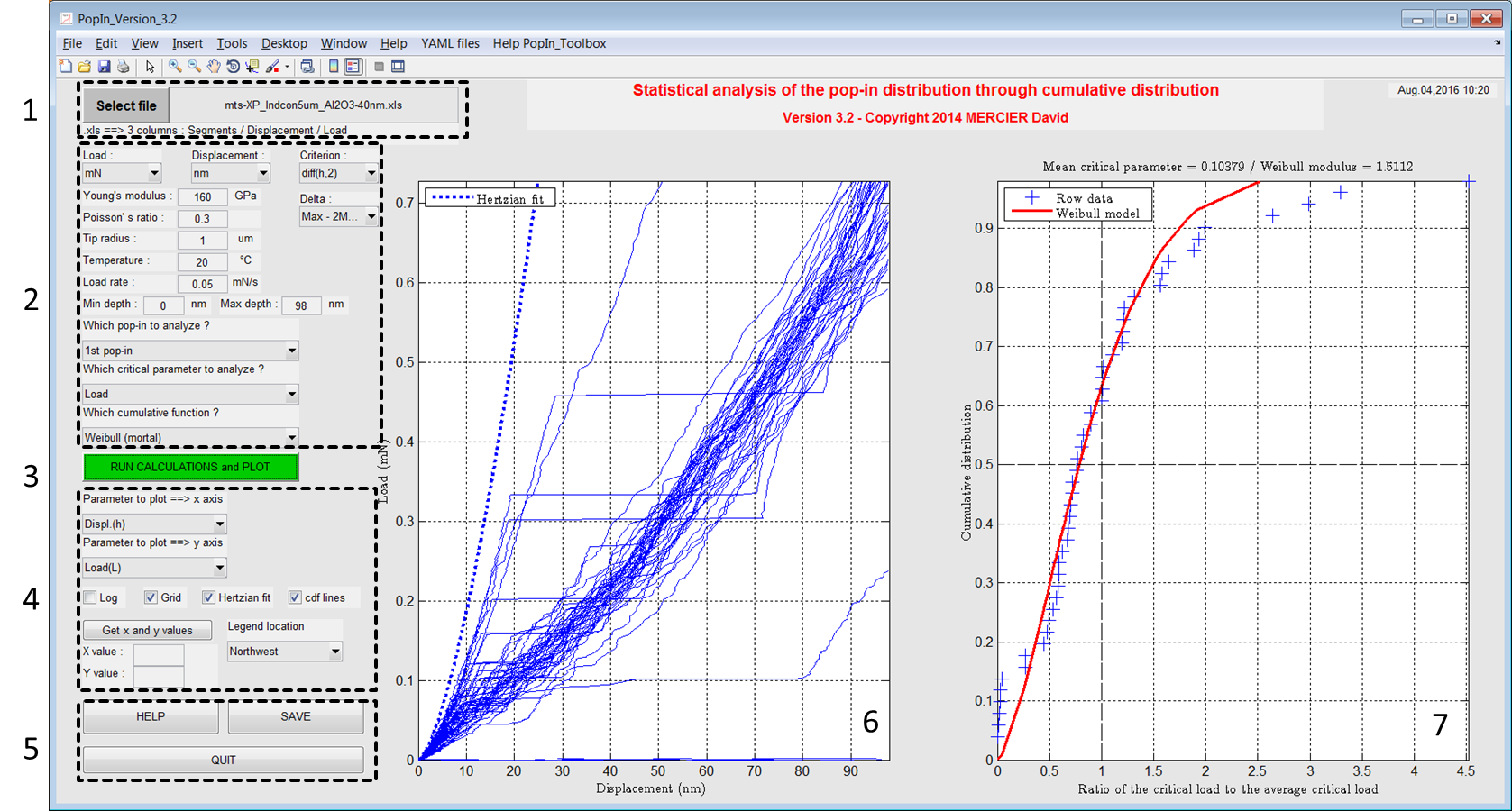# PopIn Matlab toolbox¶

This toolbox has been developed to plot (nano)indentation data and to analyze pop-in events distribution.

With this Matlab toolbox, it is possible to:

• plot load-displacement curves ;
• fit the load-displacement curves with Hertz model ;
• plot cumulative (Weibull or time/temperature dependent) distribution of the 1st or the 2nd pop-in.Figure 1 Screenshot of the main window of the PopIn toolbox.

# Keywords¶

Matlab toolbox ; Graphical User Interface (GUI) ; nanoindentation ; pop-in ; cumulative distribution ; Weibull ; statistics ; load-displacement curve ; survival probability ; critical load ; critical displacement ; Hertz model.